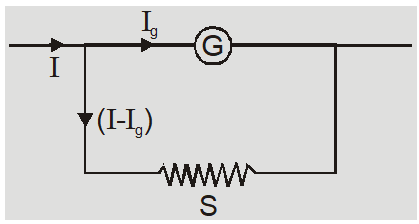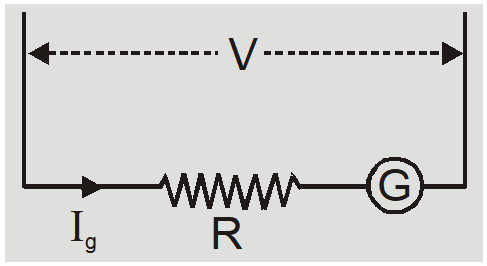Download the eSaral app and start learning from Kota's top IITians and doctors.

# Ammeter and Voltmeter Circuit Diagram | Current Electricity Class 12, JEE & NEET

Voltmeters and ammeters are used to measure voltage and current, respectively. Here we will discuss both with Ammeter and Voltmeter Circuit Diagram.

## Ammeter

1. An ammeter is a low resistance galvanometer used to measure strength of current in an electrical circuit.
2. An ammeter is always connected in series in a circuit because when an ammeter is connected in series it does not appreciably change the resistance of circuit and hence the main current flowing through the circuit.
3. In ideal ammeter has zero resistance.
4. The reading of an ammeter is always less than actual current in the circuit because all practical ammeters have low finite resistance.
5. Smaller is the resistance of an ammeter more accurate will be the reading.
6. A galvanometer can be converted to an ammeter by connecting a low resistance shunt in parallel to coil of galvanometer. Ammeter Circuit Diagram:Here $\mathrm{I}_{9} \mathrm{G}=\left(\mathrm{I}-\mathrm{I}_{\mathrm{g}}\right) \mathrm{S}$ or $\quad S=\frac{I_{g}}{I-I_{g}} G$ Here G is resistance of galvanometer and $I_{g}$ is current required to produced full scale deflection of current.
7. Shunt (S) : It is a low resistance connected in parallel to coil of galvanometer to convert it to ammeter. It protects a galvanometer from strong currents. It is also used to alter range of an ammeter.
8. The resistance of converted ammeter is $R_{A}=\frac{G S}{G+S}$
9. The range of an ammeter is increased by reducing shunt resistance S. If $\mathrm{I}=\mathrm{N} \mathrm{I}_{\mathrm{g}}$ then $S=\frac{I_{g}}{N I_{g}-I_{g}} G=\frac{G}{N-1}$ The range of an ammeter can be increased N times by reducing shunt to S = G/N-1.
10. It is possible to increase the range of an ammeter because it lowers the resistance of ammeter further.
11. The length of the shunt required $\ell=\pi r^{2} S / \rho$ where r is radius of shunt wire and  is specific resistance of material of shunt wire.
12. Reducing the shunt resistance may increase range but it reduces the sensitivity.

## Voltmeter

1. A voltmeter is a high resistance galvanometer used to measure potential difference.
2. A voltmeter is connected in parallel to a circuit element because when connected in parallel it draws least current from the main current. So it measures nearly accurate potential difference.
3. An ideal voltmeter has infinite resistance.
4. The reading of a voltmeter is always less than actual value because all practical voltmeter may have large but finite resistance.
5. Greater is the resistance of voltmeter more accurate is its reading.
6. A galvanometer is converted to a voltmeter by connecting a high resistance in series with coil of galvanometer. $V=I_{g}(R+G) \quad$ or $\quad R=\frac{V}{I_{g}}-G$7. The resistance of converted voltmeter is $\mathrm{R}_{\mathrm{v}}=\mathrm{R}+\mathrm{G}$
8. The range of a voltmeter is increased by increasing the series resistance. If $\quad \mathrm{V}=\mathrm{NV}_{9}=\mathrm{NI}_{9} \mathrm{G} \quad$ then $\quad R=\frac{N I_{9} G-G}{I_{9}}=(N-1) G$ The value of resistance required to increase range $N$ times is $R=(N-1) G$

Ex. What is the value of shunt which passes 10% of main current through a galvanometer of $99 \Omega ?$ Sol.

$S =\frac{ I _{ g } G }{ I - I _{ g }}$

where $I_{9}=\frac{10}{100} I=0.1 I$

So $S=\frac{0.1 I \times 99}{I(1-0.1)}=\frac{9.9}{0.9}=11 \Omega$.